# Difference between revisions of "MediaWiki:Sidebar"

Let <html>be a random parameter with an a priori density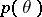, letbe a random result of observations and letbe the conditional density ofwhen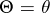; then the a posteriori distribution offor a given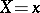</html>, according to the Bayes formula, has the densityIf <html>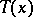</html> is a sufficient statistic for the family of distributions with densities <html>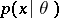, then the a posteriori distribution depends not onitself, but on. The asymptotic behaviour of the a posteriori distributionas, whereare the results of independent observations with density,</html> is  "almost independent"  of the a priori distribution of <html></html>.